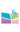﻿2023.09.22，杨雄锋，教授，上海交通大学，Hydrodynamic limit of Boltzmann equations for mixture gas-中国矿业大学（北京）理学院网站2023.09.22，杨雄锋，教授，上海交通大学，Hydrodynamic limit of Boltzmann equations for mixture gas

In this talk, I will present the hydrodynamic limit of Boltzmann equations for mixture gas in the whole-space. Precisely, we derived the compressible Euler equations for two-component fluid from the kinetic system with molecular potential $-3/2<\gamma \leq 1$ by Hilbert expansion, then we justified the validity of this expansion under the assumption of the local existence of the smooth solution for compressible Euler equations. Our approach is based on the framework established in (Y. Guo, J. Jang and N. Jiang Comm. Pure Appl. Math. 63 (2010) no. 3, 337-361).